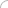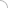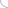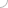# Probability - aptitude test

Home > Aptitude test > Probability

### 1) Three unbiased coins are tossed. What is the probability of getting at least 2 tails?

a) 0.75
b) 0.5
c) 0.25
d) 0.2

Solution :

S = {HHH, HHT, HTH, HTT, THH, THT, TTH, TTT}
E = {HTT, THT, TTH, TTT}
n(S) = 8
n(E) = 4
P(E) =n(E) / n(S) = 4/8 = 0.5

### 2) Tickets numbered 1 to 50 are mixed and one ticket is drawn at random. Find the probability that the ticket drawn has a number which is a multiple of 4 or 7?

a) 9/25
b) 9/50
c) 18/25
d) None of these

Solution:

S = {1, 2, 3, … , 49, 50}
E = {4, 8, 12, 16, 20, 24, 28, 32, 36, 40, 44, 48, 7, 14, 21, 35, 42, 49}
n(S) = 50
n(E) = 18
P(E) = n(E)/n(S) = 18/50
= 9/25

### 3) From a pack of 52 cards, one card is drawn at random. Find the probability that the drawn card is a club or a jack?

a) 17/52
b) 8/13
c) 4/13
d) 1/13

Solution:

n(S) = 52
n(E) = 16
P(E) = n(E) / n(S) = 16/ 52
= 4/13

### 4) Two friends A and B apply for a job in the same company. The chances of A getting selected is 2/5 and that of B is 4/7. What is the probability that both of them get selected?

a) 8/35
b) 34/35
c) 27/35
d) None of these

Solution:

P(A) = 2/5
P(B) = 4/7
E = {A and B both get selected}
P(E) = P(A)*P(B)
= 2/5 * 4/7
= 8/35

 Discussion Board Aptitude Sir your way of explanation is just awesome I cleared all the doubts Javed 07-12-2021 12:45 PM Probability A bag contains 7 white balls and 4 red balls. Five balls are drawn from it. Find the probability of getting 3 white and 2 red balls. Ashutosh 05-31-2021 09:43 AM Probability Please Solve the belowKevin wants to buy 11 balloons. In store plain balloon costs \$0.75, Multicolor bolloon costs \$1.30 and extra large bolloon costs \$1.50. How many different combinations of 11 balloons can kevin buy out of \$12. Rajani 02-10-2021 01:13 AM pls teel me the ans of this question There are 4 baskets. The first basket has 3 apples and 4 oranges, the second one has 4 apples and 5 mangoes, the third one has 6 Mangoes and 2 bananas and the last one has 7 bananas and 2 apples. If a fruit is randomly chosen from any basket and it comes out to be an apple, then what is the probability that it was taken out from the second basket? Rishav Anand 09-20-2020 11:12 AM Problem on probability Sir, could you please comment on the following problem:12 coins are to be distributed among 5 beggars, A,B,C,D and E. Find the probability that (i) They get 4, 2, 0, 5 and 1 respectively.(ii) Each beggar get at least 2 coins.(iii) None of them goes empty handed. vikas 04-24-2020 03:41 PM Probability sir could you please explain me the below problem A box contains 10 bulbs,of which just three are defective. If a random sample of five bulbs is drawn, find the probability that the sample contains exactly one defective bulb. Niveditha Nirmala 07-7-2019 01:57 AM need examples sir i need examples tahir jagroo 10-24-2018 09:59 AM PROBABLITY SIR YOUR WAY OF EXPLINATION IS AMAZING IN VIDEO TUTORIAL THANKING FOR FREE LECTURES A.MOHAN SHARMA 07-11-2018 11:28 AMAptitude questions